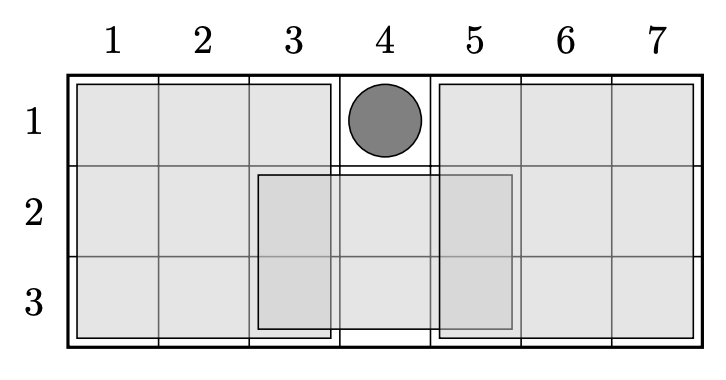시간 제한 메모리 제한 제출 정답 맞은 사람 정답 비율
1 초 512 MB 0 0 0 0.000%

## 문제

This time Byteasar researches a wildlife in a nature reserve that has a shape of an $X \times Y$ rectangle. It is divided into $XY$ unit squares, there is a square with coordinates $(x, y)$ for every $1 \le x \le X$ and $1 \le y \le Y$.

Our hard-working researcher distinguished $n$ species of animals and discovered that each species dislikes living on some particular rectangle (which is stricly smaller than whole nature reserve). For species number $i$ it is rectangle described by its two opposite corners $(x_i, y_i)$ and $(x'_i, y'_i)$, for some $x_i \leq x'_i$ and $y_i \leq y'_i$. We know that there are $c_i$ animals in that species. Therefore, there are $S = c_1 + c_2 + \ldots + c_n$ animals in total.

Byteasar has an idea for a social-natural experiment which relies on putting each of $S$ animals in some cell outside of its disliked region. Sociality of a placement is a number of pairs of animals so that both of them are in the same cell. Hence, if a cell contains $p$ animals, this adds $\frac{p(p-1)}{2}$ to the overall sociality.

It is allowed to put animals from the same species into different cells.

Find the maximum value of the sociality that can be attained.

## 입력

The first line of input contains three integers $n$, $X$ and $Y$ ($1 \leq n \leq 100\,000$, $1 \leq X,Y \leq 1000$) denoting the number of species and dimensions of nature reserve, respectively.

Each of following $n$ lines contains a description of species, $i$-th of them contains five integers $x_i, y_i, x'_i, y'_i, c_i$ ($1 \leq x_i \leq x'_i \leq X$, $1 \leq y_i \leq y'_i \leq Y$, $1 \leq c_i \leq 1000$) describing region disliked by species number $i$ and number of animals in that species. For each $i$ at least one of the following conditions holds: $x_i \neq 1$, $y_i \neq 1$, $x'_i \neq X$, $y'_i \neq Y$

## 출력

You need to print one integer -- the maximum possible sociality of some placement.

## 예제 입력 1

2 1 2
1 1 1 1 3
1 2 1 2 4


## 예제 출력 1

9


## 예제 입력 2

3 7 3
1 1 3 3 1
5 1 7 3 1
3 2 5 3 1


## 예제 출력 2

3


## 힌트

In first sample we need to put four animals in a cell $(1, 1)$ (contributing $\frac{4 \cdot 3}{2} = 6$ to the sociality) and put three remaining animals in a cell $(1, 2)$ (contributing $\frac{3 \cdot 2}{2} = 3$ to the sociality).

Second sample test is depicted below. All animals can be put in a cell $(4, 1)$.